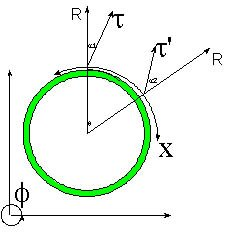# HyperGeometrical Universe TopologyHyperGeometrical Cosmology

The HyperGeometrical Universe theory is a simple Theory of Everything that based on clues from Relativity and Quantum Mechanics proposes that the Universe is actually an expanding shell on a light-speed expanding hyperspherical space disturbance. That shell where our Universe lies is a three dimensional hyperspherical shell that travels outwards at the speed of light.

Simple inspection of the above figure allows for many conclusions.

1. The first one is the Hubble expansion and why the Hubble constant is not a constant.
2. The Big Bang occurred in all points of the Universe at the same time. This explains why wherever you look, if you look far enough, you will see the beginning of the Universe.
3. In a three dimensional Big Bang a tremendous amount of anisotropy would ensue. One does not expect to find pieces of a bomb where the bomb exploded. Many "explanations" were proposed but as far as I can tell, they were not palatable.
4. The Four-Dimensional Big Bang is the only model that allows for a smooth microwave cosmic background. It is my believe that the background is actually the Doppler shifted gamma radiation from the early few seconds after the Universe was set in motion.
5. The reason for 2) is that at the time the Universe was set in motion (point of supersymmetry of the hyperforce), energy flew from each and every point of the early Universe. As the Universe expanded, energy that traveled the dimensional age of the Universe (circa 15 Billion Light years) will be reaching you by now no matter where you look.
6. There is a funny corollary: Everyone is the center of the Universe (at least of the three-dimensional shell where we live).
7. Newton proposed that a body under the influence of no force will travel in straight line with constant velocity. This model explains why it will continue moving and inspection of the figure above shows that is is never a straight line.
8. The HyperGeometrical Universe Theory shows that non-inertial motion takes place through the change in propagation vector. Two radial trajectories (outgoing along the Radial fourth-dimension or k-vector along the Radial direction) at positions separated by alpha radians (see figure above) will run away (Hubble expansion) from each other at speed alpha * c (velocity of light). If a body starts moving from Point A with speed alpha*c, it will continue moving until it reaches a point alpha radians from Point A (Point B). Reaching that Point B the body will simply move outwards while its Hubble speed from Point A will remain alpha*C. In this model , motion is due to a change in k-vector which has a dual equivalent as a local spacetime deformation. The motion from point A to point B relaxes spacetime and make it naturally curved again. This means that the HyperGeometrical Universe Theory provides the reason for free motion and complements Newton's Law...:)
9. Of course, inspection of the figure also indicates that opposing point in the Universe will travel apart at speed equal to Pi*C (greater than the speed of light). Of course, this is immaterial since it is impossible to cross more than a radian in any direction (at the speed of light through the duration of the Universe).
10. Two regions of the shell separated by one radian will run away from each other at the speed of light...
11. If you look into the sky at positions distant from you the dimensional age of the Universe, you will be looking at your very next neighboring point when the Universe was just a few millimeters wide.
12. In summary, the Universe has a radius of curvature equal to the dimensional age of the Universe, is finite and circular and cannot ever be traversed.
13. The answer to the Age Old question of what do we have after the end of the Universe is more Universe (you just cannot go there ever..:)
14. The Universe is in expansion and that doesn't depend upon the Universe mass content nor Dark Energy or anything slightly shadowy... This is an expanding four-dimensional Universe. Einstein could only see three dimensions and thus was concerned with the mass content of the Universe and the mass induced curvature. In this model, these kind of considerations might have a meaning locally.
15. The average curvature is defined by HyperGeometry (or Geometry)...
16. Please review the attached paper HyperGeometrical Universe - Cosmology and the original HyperGeometrical Universe -Grand Unification and feel free to ask any questions or to provide any comments.
Currently unrated

### New Comment

required

required (not published)

optional

required